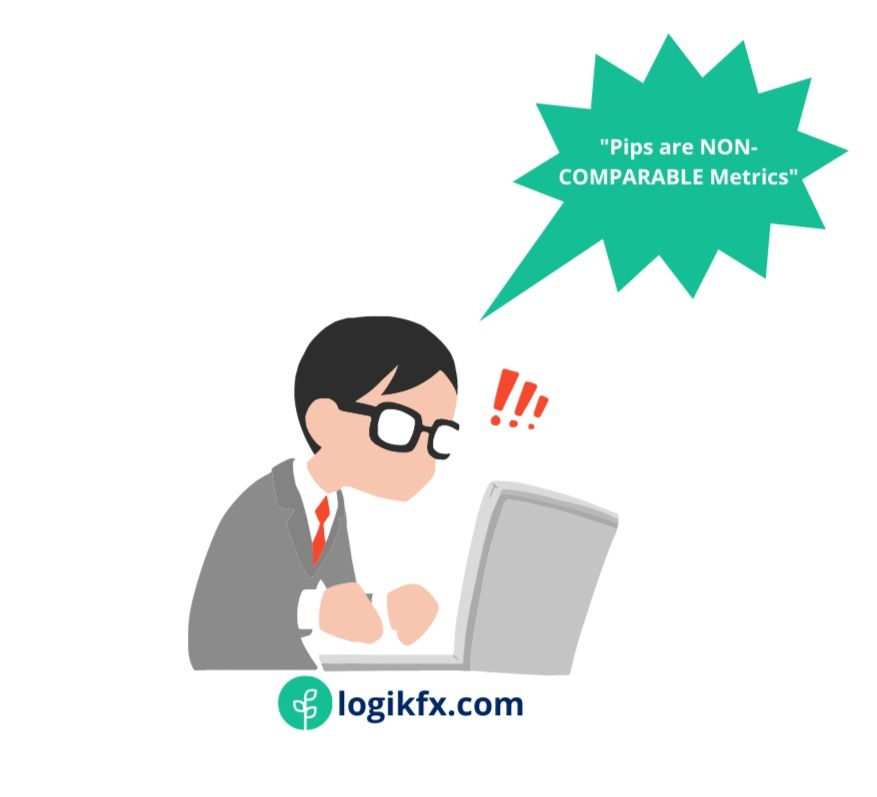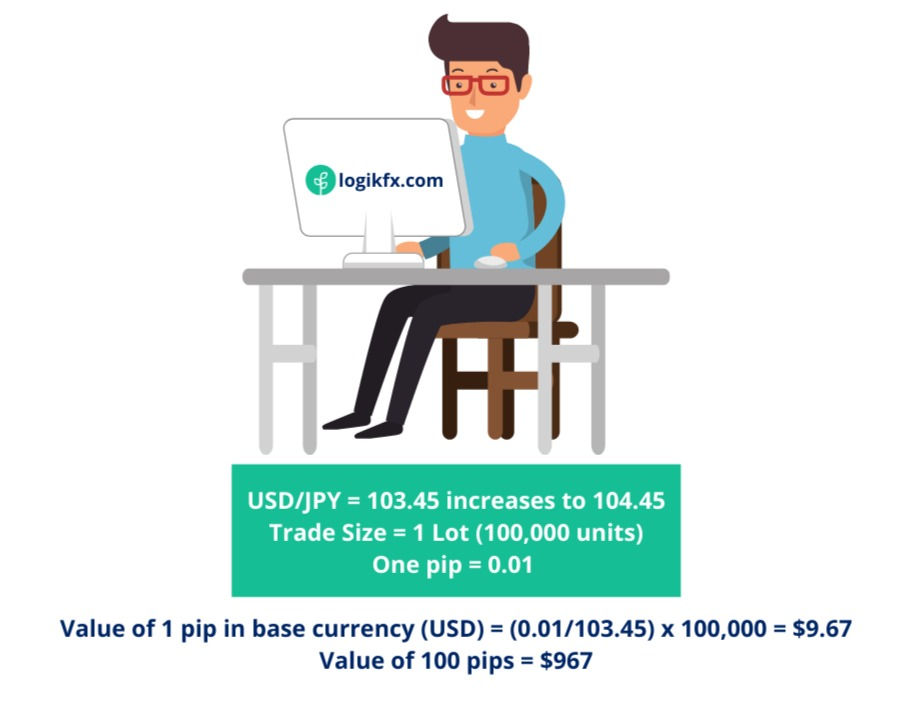top of page

# What is a Pip?

Updated: Jul 8, 2021

## Summary of Forex Pips

Pips, also known as "percentage in point" or "price interest point" are a popular form of measuring trading performance in the retail scene. As you may already know, just because it's popular, does not mean it's the best measurement to use.

• What is a pip?

• Why do traders use pips?

• How are pips calculated?

• The major flaws of using pips.

• Debunking the myth that a positive pip count means you're a profitable trader.

• The BETTER alternative to pips used in professional environments.

• The Ultimate Basis Point Calculator

By the end of this article, you should be a "Pip Connoisseur" and be able to show-off to your friends with your expert knowledge.

Let's get into it...

## 1. What is a pip?

I guess this would be your first question, well, let me explain, a pip is the term used to measure the smallest price movement in a given exchange rate.

In Forex, pretty much all currency pairs are quoted to 4 decimal places, and the "pip" is the name of the that fourth decimal point.

In other words:*This is only for non-JPY currency pairs.

Naturally then:

• 10 pips = 3.d.p

• 100 pips = 2.d.p

• 1,000 pips = 1.d.p## 2. Why do traders use pips?

This is a very fair question. The only answer really is to make their lives easier when they're talking about their positions to their friends and colleagues.

Nobody has the time to say:It just would sound a bit silly. Imagine the news anchors on Bloomberg TV having to vocalise market changes the long winded way each time... it would instantly alienate the watchers!

So, instead they will use pips, and the above example would simple read,

"My EUR/USD Trade has moved by 5 pips"

Much more pleasing to the ears. Now we know why pips are used, let's see how they're calculated.

Pssst! this is where you'll discover the major FLAW of using pips... so pay close attention

## 3. How are Pips Calculated?

Pips are calculated by MULTIPLYING the change in the exchange rate by 10,000 (excluding Japanese Yen Pairs). So for example, If GBP/USD moves from 1.3240 up to 1.3241 that is the same as saying:

GBP/USD has increased by 0.0001

Now if we multiply that change by 10,000 we'll discover how many pips it's moved:

0.0001 x 10,000 = 1 pip move on GBP/USDMost forex pairs will show 4 numbers after the decimal place; however, Japanese Yen pairs are calculated slightly differently... instead of the 4th d.p. being representative of a pip, on Yen pairs it's the 2nd d.p.

Therefore on currency pairs such as EURJPY and USDJPY we would multiply the change in price by 100.

For example, if USDJPY moves fromThis is where the problems start arise. If 1 pip on USDJPY is 0.01 of a move, and 1 pip on GBPUSD is 0.0001 pip of a move... it's a non comparable metric.Simply put, if you're running a portfolio, and you're measuring all your results in pips, it will heavily skew how well you're actually performing... to the point where it's actually useless using pips at all.

Before I can prove that to you, I must first show you how to calculate the monetary value of pips on each trade.

## 4. How to calculate the VALUE of a pip

Now we know what 1 pip means, how do we know what 1 pip is worth to us when we trade?

Not all pips are created equal. 1 pip could be £10, £100, £1,000... it all depends on the position size that you open.

Just like snowflakes every pip calculation is unique to:Now all you need to do is divide (2) by (1), then multiply by (3). This means, due to variations in exchange rates, the value of a pip will change dependant on what pair you're trading!

Top tip:

When the counter (quote or 'right-hand side') currency is the U.S. dollar, the value of a pip is always the same.

E.g. if the lots size is 10,000, the pip will equal \$1. If the lot size is 100,000 the pip will equal \$10.• one pip = 0.01 (on JPY pairs), 0.0001 (on major pairs)

• base currency = currency on the 'left-side' of the pair

• Exchange rate = Exchange rate quote for the pair you're trading

• Position Size = The amount of units you're trading. E.g. 100,000 units (1 Lot), 10,000 units (Mini Lot or 0.1 Lots), 1,000 Unit (Micro Lots or 0.01 Lots)

Let's take a practical example to see how this works:Now, if your trading account is denominated in USD, you'll want to know what each of your pips is worth in USD. How do we do this?

Well, if we know the value in Australian Dollars is approximately 1.4/ pip and the exchange rate of AUD/USD is 0.7134 - we would want to multiply the exchange rate by 1.4.

Why?

Think of AUD/USD as a ratio, where 1 Aussie dollar, buys you 0.7134 U.S dollars. Now if 1 pip is worth 1.4 Aussie dollars, we multiply the ratio of U.S. dollars by this number to get the value in U.S. dollars.And this confirms the above top tip above. If the counter currency is USD, 10,000 units will always means a movement of 1 pip is \$1.

## 5. Having positive pip gains, does not mean you're profitable!

The best way to demonstrate the biggest problems of "pips" is with a simple example:

On the first day of the week, Charles decides to trade USD/JPY Long, and he makes 100 pips. His position size was 1 Lot. How much Money did he make?The next day Charles decides to Short EUR/USD and loses 98 pips. How much money did he lose?Charles is now sitting at his trading desk, calculating how well he performed over the past couple days, and notices something quite unusual...So even though, he had traded the same position size of 10,000 units, and had a positive pip count, he still lost money.

This is why professional traders never use pips as a metric to measure their return. Instead, they use a base metric.

## 6. What is a base metric?

A base metric is a measurement that is universal for the items you're measuring. Examples include:

• Centimetres (CM)

• Miles (m)

• Ounces (Oz)

• Etc...

In trading we're measuring the change in asset prices and portfolio performance... so unfortunately 'cm' just wouldn't cut it. Can you guess the best base metric to use in trading?

Percentages!

Percentages are the universal measuring tool for changes in price. But shouting out 0.0001% change isn't quite attractive to say.

Instead, professional traders use something called... Basis Points, or Bips for short.

## 7. The BETTER alternative to pips: Basis Points (Bips)

The Basis Point, also referred to as "bips" or "bps" that describes a percentage change in the price of an asset.

In the trading world, bips are superior to 'pips' due to the fact that they mean the same thing for every single asset in existence. This means running a portfolio with forex majors, minors, stocks, bonds, indexes... is much easier to keep track of.

Alternatively, if traders had trades in stocks and forex, and measured all their recordings in pips... they would have no clue whether they're making money or not! #rookiemistake

## 8. What is a basis point (Bip)?

One basis point is equivalent to 1/100th of a percent, or in other words 0.01%. Likewise 2.5 bips is equivalent to 0.025%.## 9. How are Bips (Basis Points) Calculated?

The best way to learn is with an example!

Martin decides to open a Long Trade on GBPNZD at the current exchange rate of 1.4523. After a couple days the exchange increases to 1.4653. What would the increase be in terms of bips?

Step 1

The first step is to calculate the percentage change in price. You work this out by minusing the previous price from the current price, then divide the result by the previous price and multiply by 100:• Current price = The price the exchange rate is currently

• Previous price = The price at which the exchange rate was previously (when the trade opened)

Step 2

The second step to solving Martin's problem is to multiply our answer by 100.0.89% * 100 = 89 bps

## 10. How to use the Basis Point (Bip) Calculator## IT’S THAT SIMPLE FOLKS!

Here at Logikfx we pride ourselves on our unique trading style, being able to emulate the practices and therefore the success of professional traders.Basis points are one way that we do this, you see, Brokers and other educators will quote everything in pips to confuse you as every asset has a different pip value.

With bips everything is transferred onto a level playing field allowing you to accurately compare and asses currency pairs volatility for the best trading experience.

Professional Traders, Asset Managers and Hedge Fund Managers all use basis points when referencing their assets performance and don’t fall for the nonsense of pip talk.

“The unit size of forex pairs in the currency markets is calculated in pips, but do not make the mistake that opportunity and risk should be calculated in pips.” - CEO, Marcus Raiyat

Still learning how to trade? Learn through Logikfx Investment and Trading Academy (LITA) and take the first steps into growing your value as a trader with our free online courses, webinars, seminars. All from a small team of highly skilled traders with over 15 years’ experience in the financial markets. Learn how to make money trading forex, alongside the best ways to manage your risk through a proper trading journal, and sensible approaches to setting a stop loss (that doesn't get hit)!

Already know how to trade? Save hundreds of hours each month on trading technology, analysis and research using Logikfx's Macro Technology in the LITA Portal. Computing thousands of fundamental reports for over 23 economic regions, you'll know accurate currency strength at the click of a button.

825 views

See All